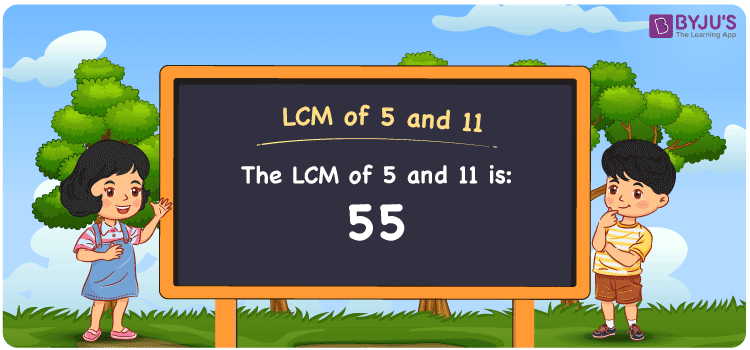Checkout JEE MAINS 2022 Question Paper Analysis : Checkout JEE MAINS 2022 Question Paper Analysis :

# LCM of 5 and 11

LCM of 5 and 11 is 55. The LCM value is the number which is divisible evenly by the given numbers 5 and 11. From the common multiples of 5 and 11, the least common multiple of 5 and 11 can be determined. The multiples of 5 are (5, 10, 15, 20, 25, ….) and the multiples of 11 are (11, 22, 33, 44, 55, ….) respectively. Prime factorisation, division and listing of the multiples are the common methods to get the LCM value.

## What is LCM of 5 and 11?

The answer to this question is 55. The LCM of 5 and 11 using various methods is shown in this article for your reference. The LCM of two non-zero integers, 5 and 11, is the smallest positive integer 55 which is divisible by both 5 and 11 with no remainder.## How to Find LCM of 5 and 11?

LCM of 5 and 11 can be found using three methods:

• Prime Factorisation
• Division method
• Listing the multiples

### LCM of 5 and 11 Using Prime Factorisation Method

The prime factorisation of 5 and 11, respectively, is given by:

5 = 5¹

11 = 11¹

LCM (5, 11) = 55

### LCM of 5 and 11 Using Division Method

We’ll divide the numbers (5, 11) by their prime factors to get the LCM of 5 and 11 using the division method (preferably common). The LCM of 5 and 11 is calculated by multiplying these divisors.

 5 5 11 11 1 11 x 1 1

No further division can be done.

Hence, LCM (5, 11) = 55

### LCM of 5 and 11 Using Listing the Multiples

To calculate the LCM of 5 and 11 by listing out the common multiples, list the multiples as shown below

 Multiples of 5 Multiples of 11 5 11 10 22 15 33 20 44 25 55 30 66 35 77 40 88 45 99 50 110 55 – 60 –

LCM (5, 11) = 55

## Video Lesson on Applications of LCM## LCM of 5 and 11 Solved Examples

If the product of two numbers is 55, what is the LCM if the GCD is 1?

Solution:

It is given that;

GCD = 1

Product of two numbers = 55

We know that,

LCM x GCD = product of two numbers

LCM = product of two numbers/ GCD

LCM = 55/1

LCM = 55

Hence, the LCM is 55.

## Frequently Asked Questions on LCM of 5 and 11

### What is the LCM of 5 and 11?

We know that,

Multiples of 5 = 5, 10, 15, 20, 25, ….

Multiples of 11 = 11, 22, 33, 44, 55, 66, ….

The smallest multiple exactly divisible by 5 and 11 is 55.

### If the LCM of 5 and 11 is 55, determine the GCF.

LCM x GCF = 5 x 11

Given

LCM of 5 and 11 is 55

55 x GCF = 55

GCF = 55/55 = 1

### What are the methods used to find the LCM of 5 and 11?

The methods used to find the LCM of 5 and 11 are

Division method

Prime Factorisation

Listing the multiples